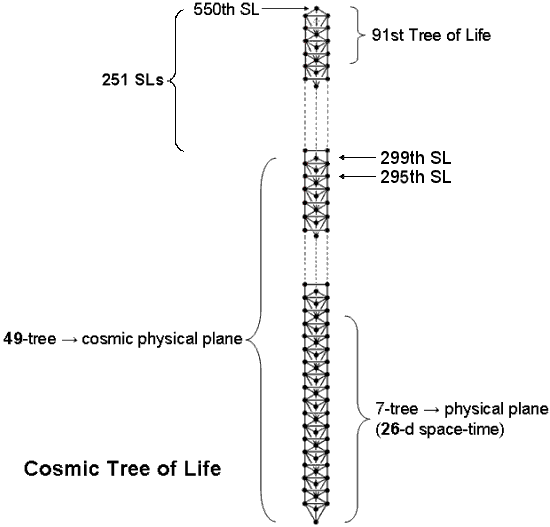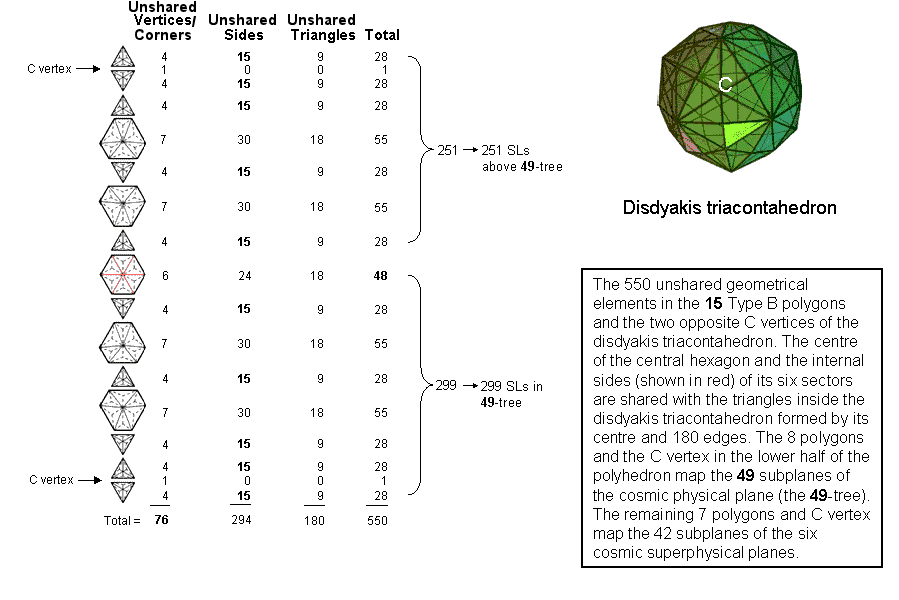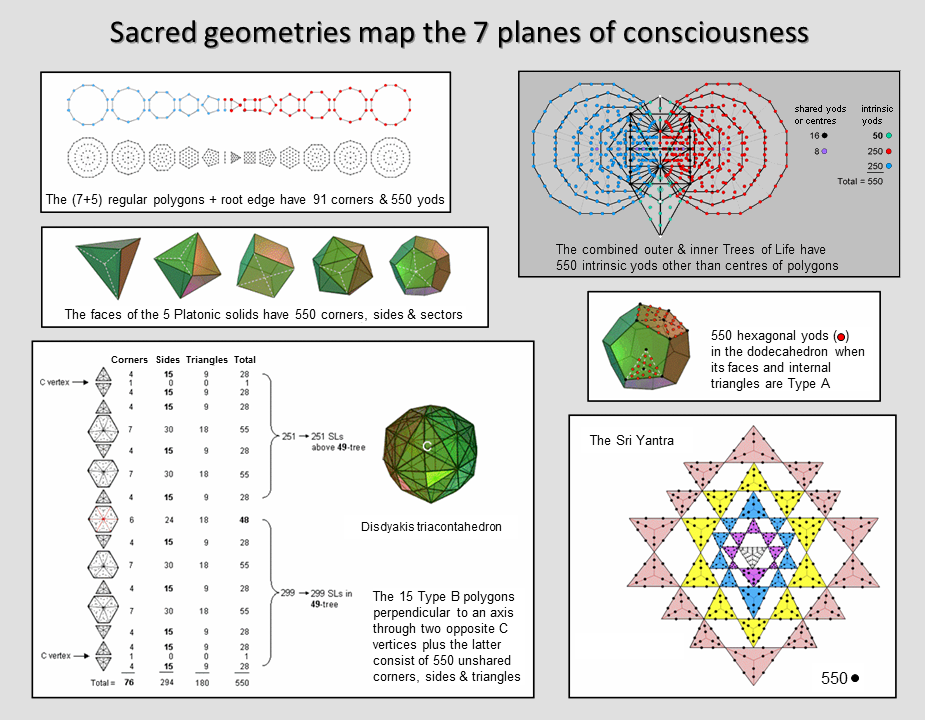Home

The disdyakis triacontahedronThe polyhedral counterpart of the Cosmic Tree of Life

The table displays the 15 polygons formed by the 60 vertices of the disdyakis triacontahedron that surround an axis passing through two opposite C vertices (vertices of a dodecahedron). They are discussed further in #11 and #12 at Sacred geometry/Polyhedral Tree of Life. When they are Type B polygons, the centre of the disdyakis triacontahedron is surrounded by 76 points that are either corners of triangles inside polygons or these two C vertices — all of which are unshared with both polyhedron and polygons. This shows how YAHWEH ELOHIM, the Godname of Tiphareth with number value 76, prescribes this pattern of points. The six red internal sides of the central hexagon are also sides of other internal triangles generated by joining the centre of the polyhedron to its vertices. The sharing applies only to this particular hexagon because none of the other polygons lies in the planes of internal triangles with the centre of the polyhedron and its vertices as their corners. None of the sides of the 15 polygons are edges of the disdyakis triacontahedron (see #11 & #12 at Sacred geometry/Polyhedral Tree of Life). The second column indicates the number of sides of the triangles making up each Type B polygon that are unshared with the polyhedron. The central polygon is a Type B hexagon. It has 30 sides of triangles, of which (30−6=24) are unshared with internal triangles formed by joining the centre of the disdyakis triacontahedron to its vertices. ELOHA, the Godname of Geburah with number value 36, prescribes the set of 15 polygons (also prescribed by YAH, the Godname of Chokmah) because they have (76+294=370=37×10) corners & sides, where 37 is the 36th integer after 1. They also have (294+180=474) sides & triangles, where 474 is the number value of Daath. The number of corners/vertices & triangles = 76 + 180 = 256 = 44, showing how the Pythagorean Tetrad expresses this property. The table shows that the centre of the disdyakis triacontahedron is surrounded by 550 geometrical elements in the 15 polygons and the polyhedron that are unshared with one another. This number is the number of SLs in the Cosmic Tree of Life! We discover the remarkable fact that each unshared geometrical element making up the disdyakis triacontahedron and the 15 polygons formed by its vertices corresponds to an SL of CTOL! As the root edge of the two sets of seven polygons making up the inner Tree of Life terminates at the positions of Daath and Tiphareth projected onto the plane of the (7+7) enfolded polygons, the number 550 = 474 + 76, where 474 is both the number value of Daath ("knowledge") (see here) and the number of sides & triangles and 76 is both the number value of the Godname of Tiphareth and the number of unshared corners & vertices (see also #9 at Maps of reality/Cosmic Tree of Life). The number 550, therefore, expresses "knowledge of YAHWEH ELOHIM (God the Creator)". CTOL is the domain of the manifested Divine Life, and its 550 SLs express the number of its Sephirothic modifications. EL, the Godname of Chesed with number value 31, prescribes the disdyakis triacontahedron because each half has 31 vertices. ELOHA, the Godname of Geburah, the next Sephirah after Chesed, prescribes it because a complete half of the polyhedron (including the whole central hexagon) contains 36 vertices, where 36 is the number value of ELOHA.

That it is not just a coincidence that the number of SLs in CTOL is the same as the number of unshared geometrical elements in the 15 internal polygons of the disdyakis triacontahedron is demonstrated by the facts that the eight polygons and C vertex in one half of it consist of 299 geometrical elements (see diagram above), whilst the seven remaining polygons and the other C vertex lying on the C-C axis comprise 251 geometrical elements. Amazingly, they are the counterparts of the 299 SLs in the 49-tree mapping the cosmic physical plane and the 251 SLs in the remaining 42 Trees that map the 42 subplanes of the six cosmic superphysical planes (see Maps of reality/Cosmic Tree of Life). Just as we saw in that section that one half of the inner Tree of Life encodes the 49-tree and that the five largest polygons in its other half encode the remaining 42 Trees of the Cosmic Tree of Life, so we find here that — in terms of the correspondence between geometrical elements and SLs —a physical half of the polyhedron maps the cosmic physical plane, whilst its remainder maps the six cosmic superphysical planes!  Each unshared, geometrical element making up the 15 polygons, whose planes are perpendicular to a C-C axis, corresponds to one of the 550 SLs of CTOL. The disdyakis triacontahedron is the single, polyhedral representation of the physical and spiritual cosmos. Any two diametrically opposite C vertices, which are north and south poles of the polyhedron, serve in this representation as the nadir and zenith of CTOL, namely, its first and last SLs.

The encoding of CTOL in the disdyakis triacontahedron is discussed also in #7 at Miscellaneous wonders.

The 550 geometrical elements that are unshared between the 15 polygons and the disdyakis triacontahedron are composed of 180 triangles with 76 points and 294 lines, i.e., 370 points & lines. This exactly corresponds to the geometrical composition of the 50 faces of the five Platonic solids because their 180 sectors have 370 corners & sides (see the table here). This 180:370 division manifests in the 550 hexagonal yods composing the dodecahedron as the 180 hexagonal yods within its 12 pentagonal faces and the 370 hexagonal yods that are either on its edges or in its interior (see here). The fact that three different examples of "sacred geometry" have geometrical compositions characterised by not only the same number but also its same division into two other numbers strongly refutes the possibility of coincidence. Clearly, it is extremely implausible that a number appearing supposedly by chance in three different contexts should display also by accident the same pattern of division in each case!

Established here for the first time is an indisputable, mathematical connection between the geometry of the disdyakis triacontahedron, the Theosophical doctrine of the seven cosmic planes of consciousness and their division into the 49 subplanes of the cosmic physical planes and the 42 subplanes of the six cosmic superphysical planes. Sceptics must ask themselves: how can coincidence (the only alternative, conventional explanation available to them) be a plausible reason for why the very same number 550 is naturally embodied in six sacred geometries, as demonstrated in this section of the website and as summarised in the picture shown below?:Home